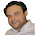Please visit, subscribe and share 10 Minutes Lectures in Computer Science

## Find minimal cover of set of functional dependencies example, Solved exercise - how to find minimal cover of F? Easy steps to find minimal cover of FDs, What is minimal cover?

Question:
6. Find the minimal cover of the set of functional dependencies given; {A → C, AB → C, C → DI, CD → I, EC → AB, EI → C}

Solution:
 Minimal cover: Definition 1: A minimal cover of a set of FDs F is a minimal set of functional dependencies Fmin that is equivalent to F. There can be many such minimal covers for a set of functional dependencies F. Definition 2: A set of FDs F is minimum if F has as few FDs as any equivalent set of FDs.

Simple properties/steps of minimal cover:
1. Right Hand Side (RHS) of all FDs should be single attribute.
2. Remove extraneous attributes. [What is extraneous attribute? Refer here].
3. Eliminate redundant functional dependencies.

Let us apply these properties to F = {A → C, AB → C, C → DI, CD → I, EC → AB, EI → C}

1. Right Hand Side (RHS) of all FDs should be single attribute. So we write F as F1, as follows;
F1 = {A → C, AB → C, C → D, C → I, CD → I, EC → A, EC → B, EI → C}

2. Remove extraneous attributes.
Extraneous attribute is a redundant attribute on the LHS of the functional dependency. In the set of FDs, AB → C, CD → I, EC → A, EC → B, and EI → C have more than one attribute in the LHS. Hence, we check one of these LHS attributes are extraneous or not.
To check, we need to find the closure of each attribute on the LHS; [apply the closure finding algorithm – refer here]
(i) A+ = ACDI
(ii) B+ = B
(iii) C+ = CDI
(iv) D+ = D
(v) E+ = E
(vi) I+ = I
From (i), the closure of A included the attribute C. So, B is extraneous in AB → C, and B can be removed.
From (iii), the closure of C included the attribute I. So, D is extraneous in CD → I, and D can be removed.
No more extraneous attributes are found. Hence, we write F1 as F2 after removing extraneous attributes from F1 as follows;
F2 = {A → C, C → D, C → I, EC → A, EC → B, EI → C}

3. Eliminate redundant functional dependency.
Here, EI → C is redundant (C can be determined using other FD, in this case, A → C). Hence, the minimal cover Fc = {A → C, C → D, C → I, EC → A, EC → B}.

Hence, set of functional dependencies Fc is the minimal cover for the set F.

**************************

Similar topics

## How to find extraneous attribute?

Go back to Question/QUIZ page

Go back to

1.2.3.If we first find redundant fds first, then C->I misses out

1.More than one minimal covers are possible

4.What about F3 = {A → C, C → D, C → I, EI → A, EI → B} ? It has only five FD's and it is equivalent to F2!

1.Yes. A set F can have several canonical cover. It depends on which FD or where do we start. Thanks for your comment.
I made a mistake. Either A-->C or EI --> C is redundant. Hence, the final Fc would be {A → C, C → D, C → I, EC → A, EC → B}

5.6.If you remove the redundancy EI -> C, how do you infer this FD back using the remaining FDs? In other words, won't we lose this dependency information if we remove EI -> C?

1.This not about inferring the FDs. It is about determining RHS attributes. We can determine C without the help of EI.

7.